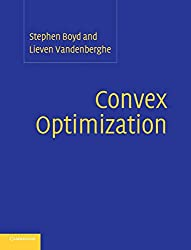# Convex optimization# Convex optimization

Convex optimization problems arise frequently in many different fields. This book provides a comprehensive introduction to the subject, and shows in detail how such problems can be solved numerically with great efficiency. The book begins with the basic elements of convex sets and functions, and then describes various classes of convex optimization problems. Duality and approximation techniques are then covered, as are statistical estimation techniques. Various geometrical problems are then presented, and there is detailed discussion of unconstrained and constrained minimization problems, and interior-point methods. The focus of the book is on recognizing convex optimization problems and then finding the most appropriate technique for solving them. It contains many worked examples and homework exercises and will appeal to students, researchers and practitioners in fields such as engineering, computer science, mathematics, statistics, finance and economics.

この本には最新版があります。この本には最新版があります。